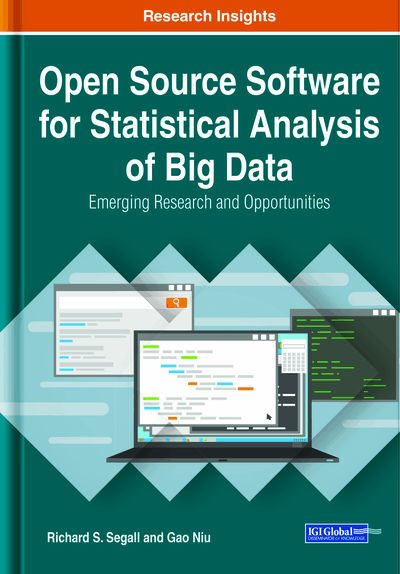# A Comparison of Machine Learning Algorithms of Big Data for Time Series Forecasting Using Python

Son Nguyen (Bryant University, USA) and Anthony Park (Bryant University, USA)
DOI: 10.4018/978-1-7998-2768-9.ch007
Available
\$33.75
List Price: \$37.50
10% Discount:-\$3.75
TOTAL SAVINGS: \$3.75

## Abstract

This chapter compares the performances of multiple Big Data techniques applied for time series forecasting and traditional time series models on three Big Data sets. The traditional time series models, Autoregressive Integrated Moving Average (ARIMA), and exponential smoothing models are used as the baseline models against Big Data analysis methods in the machine learning. These Big Data techniques include regression trees, Support Vector Machines (SVM), Multilayer Perceptrons (MLP), Recurrent Neural Networks (RNN), and long short-term memory neural networks (LSTM). Across three time series data sets used (unemployment rate, bike rentals, and transportation), this study finds that LSTM neural networks performed the best. In conclusion, this study points out that Big Data machine learning algorithms applied in time series can outperform traditional time series models. The computations in this work are done by Python, one of the most popular open-sourced platforms for data science and Big Data analysis.
Chapter Preview
Top

## Background And Literature Review

### Forecasting

Time series forecasting has largely been based around the Box-Jenkins method of Autoregressive Integrated Moving Average (ARIMA) developed in the 1960s and the Holt-Winters method of exponential smoothing developed in the 1950s. These methods, especially Box-Jenkins, rely heavily on the forecaster to visualize the time series in many ways including time plots, autocorrelation plots, and seasonal plots to find the trend, seasonality, and noise of the series before specifying the appropriate hyperparameters (also called the order). The problem with this is it takes time and experience to find the appropriate type of model and hyperparameters. Most of this process is described in Box and Jenkins’ book Time Series Analysis: Forecasting and Control, although many other sources have come out since.

Of the research that crosses over between the machine learning and time series analysis, most of them are focused on neural networks. Zhang (2002) proposed an ensemble method that uses ARIMA models to forecast the linear portion of a time series and neural networks to forecast the non-linear portion which resulted in better forecasts on three types of data. Kaastra et al. (1995) presented an eight-step process for designing a neural network for economic time series. Sharda et al. (1990), one of the most popular studies in this field, showed neural networks can perform just as well as Box-Jenkins methods for series with many observations. Although all important findings, these are not comparison papers on the effectiveness of a variety of machine learning models for time series.

The most robust research found in the cross between machine learning and time series is by Ahmed et al. (2010) where they compare multiple neural networks, KNN regressions, decision trees, and support vector machines with different preprocessing methods on the M3 economic time series data. They found that a Multilayer Perceptron and Gaussian process performed well across lagged and smoothed by rolling average data while radial basis functions resulted in poor forecasts for all preprocessing methods. The paper by Bontempi et al. (2013) also looks at multiple machine learning models for time series but focuses on reshaping the data to align with the assumptions for machine learning models. Although not a comparison paper, this is an important base of this research.

## Key Terms in this Chapter

Autoregressive Integrated Moving Average (ARIMA): A time series model that combines the differenced autoregressive model and the moving average model.

Perceptron: A binary classification model where the prediction (target) is the indicator function of the weighted sum of the inputs greater than a specified threshold.

Support Vector Machines (SVM): A classification model that compute the decision boundary that maximize the margin.

Recurrent Neural Networks (RNN): A neural network using internal state to process sequences of inputs that is commonly used in time series, natural language processing and speech recognition.

Regression Trees: A regression model uses a flowchart like tree structure. The target in the model can be expressed as a simple function of the input variables.

Exponential Smoothing Models: A time series model where the prediction of future value is a weighted sum of past values. Exponential smoothing uses exponential decreasing weight for past values.

Long Short-Term Memory Neural Networks (LSTM): A variation of Recurrent Neural Network uses feedback connection that was first proposed to solve the vanishing gradient problem when training neural networks.

Multilayer Perceptrons (MLP): A supervised model that contains multiple layers where each layer contains multiple perceptions.

## Complete Chapter List

Search this Book:
Reset